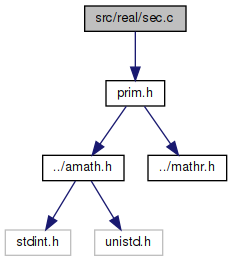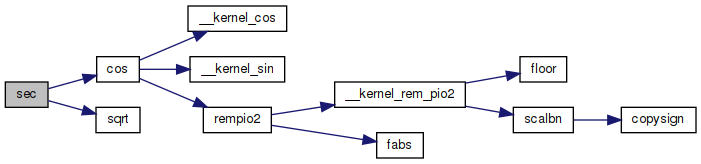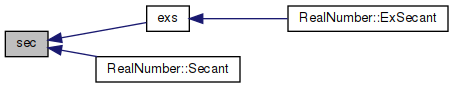amath  1.8.5 Simple command line calculator
sec.c File Reference
`#include "prim.h"`
Include dependency graph for sec.c:Go to the source code of this file.

## Functions

double sec (double x)
Secant function. More...

## ◆ sec()

 double sec ( double x )

Secant function.

```sec(x) = 1/cos(x)
= 1/sqrt(cos(x)*cos(x))
```

Definition at line 45 of file sec.c.

References cos(), and sqrt().

Referenced by exs(), and RealNumber::Secant().

46 {
47  double a, b, c;
48
49  a = cos(x);
50  if (a == 0.0)
51  {
52  return NAN;
53  }
54
55  b = sqrt(a * a);
56  c = 1.0 / b;
57
58  return c;
59 }
double cos(double x)
Cosine function.
Definition: cos.c:87
#define NAN
Definition: mathr.h:53
double sqrt(double x)
Square root function.
Definition: sqrt.c:119
Here is the call graph for this function:Here is the caller graph for this function: